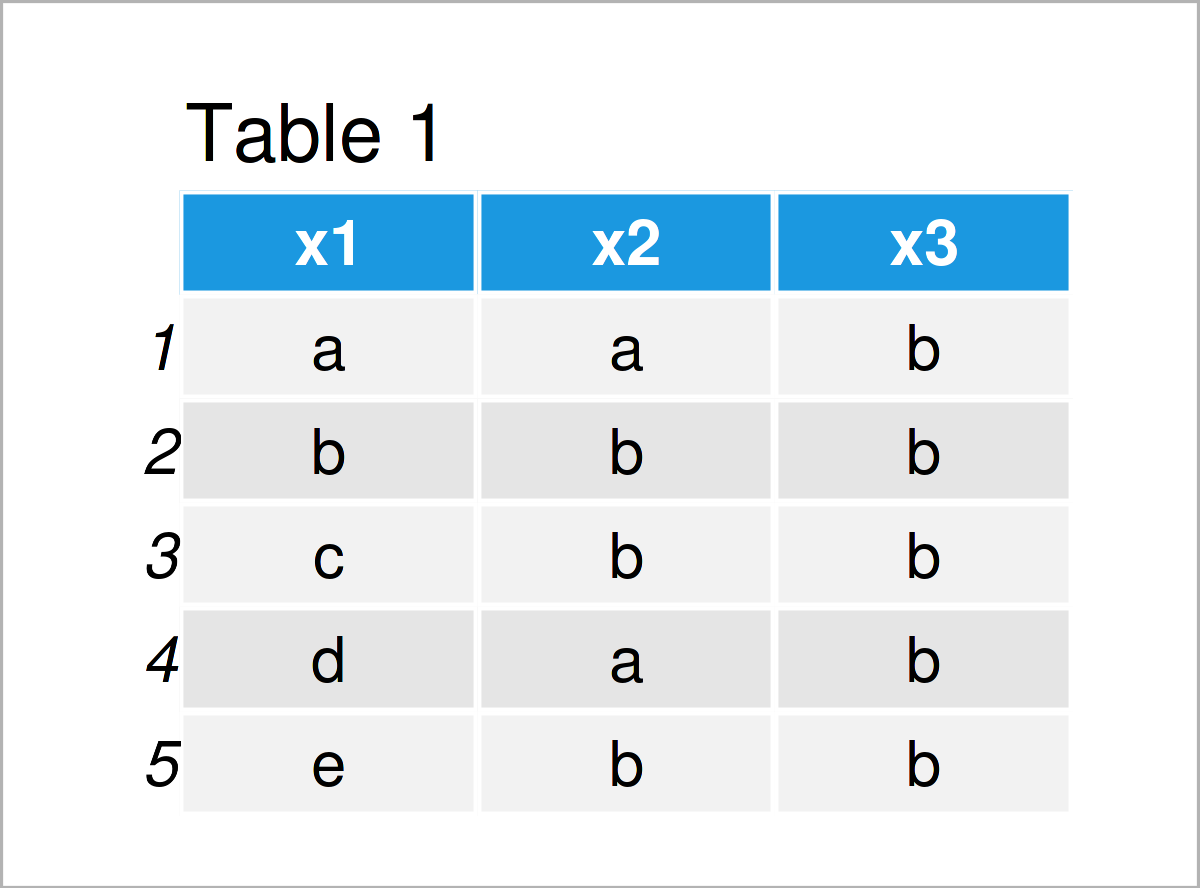# Count Occurrences of Value in Data Frame in R (2 Examples)

This tutorial explains how to count the number of times a certain entry occurrs in a data frame in the R programming language.

The post looks as follows:

Let’s take a look at some R codes in action.

## Creating Example Data

I’ll use the following data as a basis for this R programming language tutorial:

```data <- data.frame(x1 = letters[1:5],    # Create example data frame
x2 = c("a", "b", "b", "a", "b"),
x3 = "b")
data                                     # Print example data frame```Table 1 shows the structure of our exemplifying data: It is made up of five data points and three character columns.

## Example 1: Count Certain Value in One Column of Data Frame

In this example, I’ll demonstrate how to get the number of times a particular entry appears in a specific variable of a data frame.

For this task, we can apply the sum function to a logical condition as shown below:

```sum(data\$x2 == "b")                      # Count in one column
#  3```

The RStudio console has returned the value 3 after executing the previous R code, i.e. the character “b” occurs three times in the column x2.

## Example 2: Count Certain Value in Entire Data Frame

This example explains how to count a certain value in all columns of a data frame in R.

Once again, we can use the sum function to accomplish this. However, this time we are not specifying a certain column (as we did in Example 1):

```sum(data == "b")                         # Count in all columns
#  9```

As you can see, the character “b” is contained nine times in our example data set.

## Video, Further Resources & Summary

If you need further details on the R programming code of this tutorial, you might want to watch the following video that I have published on my YouTube channel. In the video, I’m explaining the examples of this article.

Besides that, you may want to read some related RStudio articles on my website. Please find a selection of related tutorials about related topics such as numeric values, data inspection, character strings, and vectors below:

To summarize: In this article, I have explained how to count a certain entry occurs in a data frame in the R programming language. Don’t hesitate to let me know in the comments section below, in case you have additional questions.

Subscribe to the Statistics Globe Newsletter

•aura
January 13, 2022 10:29 am

Many thanks.

•January 13, 2022 3:53 pm

You are very welcome Aura, glad you like it!

•zahra
January 17, 2022 2:59 pm

thanks alot👌👌👏

•January 18, 2022 1:54 pm

•Thuy Scanlon
November 28, 2022 10:42 pm

the problem with using sum(data == “b”) is that it will return NA instead of 0 if that value does not exist in the data. This is a real problem if you assume it return 0 and try to make some arithmetic calculation, especially when you write a function.

•December 14, 2022 11:30 am

Hello Thuy,

Are you sure that it is the case? Check the R script below.

```data <- data.frame(x1 = letters[1:5],    # Create example data frame
x2 = c("a", "b", "b", "a", "b"),
x3 = "b")
data

sum(data\$x2 == "b")                      # Count in one column
#  3

sum(data == "k")
#  0```

It returns 0 for the character “k”. I hope I got your point correctly. If not, please let me know.

Regards,
Cansu

•Felix D.
February 2, 2023 10:54 pm

Like Thuy Scanlon, I also got the “NA” problem.

Seems like the source of the problem is that if you sum anything with NAs present, the result will be NA, unless you handle it properly. The solution if you have NAs in your data, is to add “, na.rm=T” at the end

example: sum(data == “k”, na.rm=T)

Hope this helps!

•February 6, 2023 10:33 am

Hello Felix,

Thank you for the input, it is appreciated!

Regards,
Cansu

•Dan
September 26, 2023 3:47 pm

How would you get the value counts and percentages of all the variables in the example data frame?

•September 27, 2023 7:38 am

Hello Dan,

```df <- data.frame(
A = c('yes', 'no', 'yes', 'yes', 'no'),
B = c('blue', 'red', 'blue', 'green', 'green'),
C = c(1, 1, 2, 2, 1)
)

counts <- table(df)
counts
# , , C = 1
#
#       B
# A     blue green red
# no     0     1   1
# yes    1     0   0
#
# , , C = 2
#
#       B
# A     blue green red
# no     0     0   0
# yes    1     1   0

prop.table(counts)*100
# , , C = 1
#
#       B
# A     blue green red
# no     0    20  20
# yes   20     0   0
#
# , , C = 2
#
#       B
# A     blue green red
# no     0     0   0
# yes   20    20   0```

Best,
Cansu